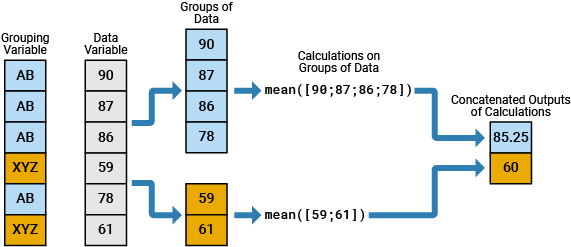# splitapply

## 语法

``Y = splitapply(func,X,G)``
``Y = splitapply(func,X1,...,XN,G)``
``Y = splitapply(func,T,G)``
``[Y1,...,YM] = splitapply(___)``

## 说明

``Y = splitapply(func,X,G)` 将 `X` 划分为 `G` 指定的组，并向每个组应用函数 `func`。`splitapply` 以数组形式返回 `Y`，其中包含对从 `X` 划分出的组应用 `func` 后的串联输出。输入参数 `G` 是由正整数组成的向量，用于指定 `X` 的对应元素属于哪个组。如果 `G` 包含 `NaN` 值，则 `splitapply` 在将 `X` 划分归组时会省略 `X` 中的对应值。要创建 `G`，您可以使用 `findgroups` 函数。`splitapply` 将拆分-应用-合并工作流中的两个步骤进行了合并。`

``Y = splitapply(func,X1,...,XN,G)` 将 `X1,...,XN` 划分归组并应用 `func`。`splitapply` 函数对每个组调用一次 `func`，同时将来自 `X1,...,XN` 的对应元素作为 `func` 的 `N` 个输入参数。`

``Y = splitapply(func,T,G)` 将表 `T` 的变量划分归组并应用 `func`。`splitapply` 函数将 `T` 的变量视为向量、矩阵或元胞数组，具体取决于表变量的数据类型。如果 `T` 有 `N` 个变量，则 `func` 必须接受 `N` 个输入参数。`

``[Y1,...,YM] = splitapply(___)` 将变量划分归组并向每个组应用 `func`。`func` 返回多个输出参数。`Y1,...,YM` 包含对从输入数据变量划分出的组应用 `func` 后的串联输出。`func` 可以返回属于不同类的输出参数，但在每次调用 `func` 时每个输出的类必须是相同的。您可以将此语法与前面语法中的任何输入参数结合使用。从 `func` 返回的输出参数的数量不必与 `X1,...,XN` 指定的输入参数的数量相同。`

## 示例

```load patients whos Gender Height```
``` Name Size Bytes Class Attributes Gender 100x1 11412 cell Height 100x1 800 double ```

`G = findgroups(Gender);`

`Height` 划分为由 `G` 指定的组。按性别计算平均高度。输出参数的第一行是女性患者的平均身高，第二行是男性患者的平均身高。

`splitapply(@mean,Height,G)`
```ans = 2×1 65.1509 69.2340 ```

```load patients whos Systolic Diastolic Smoker```
``` Name Size Bytes Class Attributes Diastolic 100x1 800 double Smoker 100x1 100 logical Systolic 100x1 800 double ```

`func` 定义为一个函数，用于计算烟民和非烟民的收缩压和舒张压血压读数之间差异的方差。`func` 要求两个输入参数。

`func = @(x,y) var(x-y);`

```[G,smokers] = findgroups(Smoker); varBP = splitapply(func,Systolic,Diastolic,G)```
```varBP = 2×1 44.4459 48.6783 ```

```numPatients = splitapply(@numel,Smoker,G); T = table(smokers,numPatients,varBP)```
```T=2×3 table smokers numPatients varBP _______ ___________ ______ false 66 44.446 true 34 48.678 ```

`mystats = @(x)[min(x) median(x) max(x)];`

`patients.mat` 加载烟民患者的体重、性别和状态信息。

```load patients whos Weight Gender Smoker```
``` Name Size Bytes Class Attributes Gender 100x1 11412 cell Smoker 100x1 100 logical Weight 100x1 800 double ```

```G = findgroups(Gender,Smoker); Y = splitapply(mystats,Weight,G)```
```Y = 4×3 111.0000 131.0000 147.0000 115.0000 131.0000 146.0000 158.0000 181.5000 194.0000 164.0000 181.0000 202.0000 ```

```load patients DT = table(Height,Weight); GT = table(Gender,Smoker);```

`meanBMIFcn = @(h,w)mean((w ./ (h.^2)) * 703);`

```[G,results] = findgroups(GT); meanBMI = splitapply(meanBMIFcn,DT,G); results.meanBMI = meanBMI```
```results=4×3 table Gender Smoker meanBMI __________ ______ _______ {'Female'} false 21.672 {'Female'} true 21.669 {'Male' } false 26.578 {'Male' } true 26.458 ```

```% Copyright 2015 The MathWorks, Inc. function [lo,avg,hi] = multiStats(x) lo = min(x); avg = mean(x); hi = max(x); end ```

```load patients T = table(Gender,Height); summary(T) ```
```Variables: Gender: 100x1 cell array of character vectors Height: 100x1 double Values: Min 60 Median 67 Max 72 ```

```[G,gender] = findgroups(T.Gender); [minHeight,meanHeight,maxHeight] = splitapply(@multiStats,T.Height,G); result = table(gender,minHeight,meanHeight,maxHeight) ```
```result = 2x4 table gender minHeight meanHeight maxHeight __________ _________ __________ _________ {'Female'} 60 65.151 70 {'Male' } 66 69.234 72 ```

## 输入参数

• 如果 `X` 为向量或元胞数组，则 `G` 的长度必须与 `X` 相同。

• 如果 `X` 为矩阵，则 `G` 的长度必须等于 `X` 的行数或列数，具体取决于 `G` 的方向。

• 如果输入参数是表 `T`，则 `G` 必须为列向量。`G` 的长度必须等于 `T` 的行数。

## 详细信息

### 拆分-应用-合并工作流